NEET and AIPMT NEET Chemistry Chemical Bonding and Molecular Structure MCQ Questions Solved

Which of the following would have a permanent dipole moment ?

(a) BF3

(b) SiF4

(c) SF4

(d) XeF4

(c)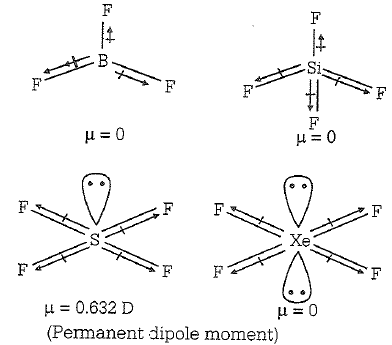Difficulty Level:

• 38%
• 16%
• 30%
• 17%

In the case of alkali metals, the covalent character decreases in the order

(a) MCl > MI > MBr > MF

(b) MF > MCl >  MBr > M

(c)MF > MCl > MI > MBr

(d) MI > MBr > MCl > MF

(d) According to Fajan's rule,

Covalent character

In the given options, cation is same but anions are different. Among halogens the order of size is

F < Cl < Br < I

$\therefore$Order of covalent character is

MI > MBr > MCl > MF

Difficulty Level:

• 10%
• 35%
• 11%
• 47%

Which of the following two are isostructural?

(a)XeF2, and IF_2

(b)NH3, and BF3

(c)CO32-, and SO32-

(d)PCl5, and ICl5

(a) Isostructural Compounds having same structure and same hybridisation are known as isostructural species. e.g. XeF2 and IF_2   are sp3d hybridised and both have linear shape.

F-I-F    F-Xe-F

Difficulty Level:

• 50%
• 21%
• 21%
• 10%

Which one of the following species does not exist under normal conditions ?

(a) ${\mathrm{Be}}_{2}^{+}$

(b) Be2

(c) B2

(d) Li2

(b) Molecules with zero bond order, do not exist.

According to molecular orbital theory,

Difficulty Level:

• 30%
• 42%
• 17%
• 13%

Which one of the following is not paramagnetic ?

(a) NO

(b) ${\mathrm{N}}_{2}^{+}$

(c) CO

(d) ${\mathrm{O}}_{2}^{-}$

(c) Paramagnetic character is shown by those atoms or molecules which have unpaired electrons.

In the given compounds CO is not paramagnetic since, it does not have unpaired electrons. The configuration of CO molecule is

CO(14) =

Difficulty Level:

• 13%
• 18%
• 55%
• 16%

In a regular octahedral molecule, MX6 the number of X-M-X bonds at 180$°$is

(a) 3

(b) 2

(c) 6

(d) 4

(a) In octahedral structure MX6, the six hybrid orbitals (sp3d2) are directed towards the corners of a regular octahedral with an angle of 90$°$. According to following structure of MX6, the number of X-M-X bonds at 180$°$ must be three.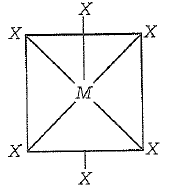Difficulty Level:

• 44%
• 32%
• 16%
• 10%

The angular shape of ozone molecule (O3) consists of

(a) 1 sigma and 2 pi-bonds

(b) 2 sigma and 2 pi-bonds

(c) 1 sigma and 1 pi-bonds

(d) 2 sigma and 1 pi-bonds

(d) In case of single bond, there is only one s-bond in case of double bond, there is one and one p-bonds. Thus, angular shape of ozone (O3) contains 2and 1 p-bonds as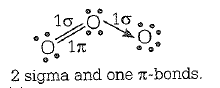Difficulty Level:

• 16%
• 17%
• 7%
• 62%

Which of the following is not isostructural with SiCl4

(a) SCl4

(b) SO42-

(c) PO43-

(d) NH4+

(a) SCl4 is not isostructural with  Sicl4 because shows square planar structure due to involvement of repulsion between lone pair and bond pair of electrons.

SO42- shows tetrahedral structure due to sp3 hybridisation.

PO43- shows tetrahedral structure due to sp3 hybridisation.

NH4shows tetrahedral structure due to sp3 hybridisation

Difficulty Level:

• 54%
• 16%
• 13%
• 19%

In which of the following molecules are all the bonds not equal ?



(a) ClF3

(b) BF3

(c) AlF3

(d) NF3

(a) ClF3 all bonds are not equal due to trigonal-bipyramidal (sp3d hybridisation) geometry of ClF3 molecule.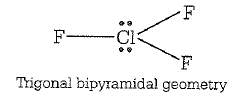BF3 and AlF3 show trigonal symmetric structure due to sp2 hybridisation.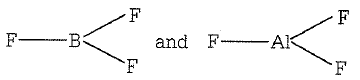NF3 shows pyramidal geometry due to sp3 hybridisation.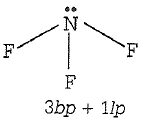Difficulty Level:

• 59%
• 14%
• 13%
• 16%

The correct order of increasing bond angles in the following species is

(a)Cl2O < ClO2${\mathrm{ClO}}_{2}^{-}$

(b) ClO2 < Cl2O < ${\mathrm{ClO}}_{2}^{-}$

(c) Cl2O < ${\mathrm{ClO}}_{2}^{-}$ < ClO2

(d) ${\mathrm{ClO}}_{2}^{-}$ < ClO2 < Cl2

(d) According to VESPR theory repulsion order

lp - lp > lp - bp > bp - bp

As the number of lone pairs of electrons increases, bond angle decreases due to repulsion between lp-lp, Moreover, as the electronegativity of central atom decreases, bond angle decreases.

Hence, the order of bond angle is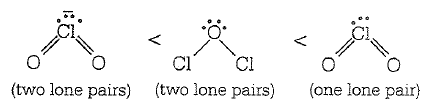(Cl is less electronegative as compared to O).

clo2- =111,cl2O ,110, Clo2 =117

explanation is given, its can be explained on the multiple concept like valence electron,hybridisation,electro negativity, but fact is ,its a experiemental science , so we need to develop theory to explain the observation, this is basis of evolution of VBT to CFT.

Difficulty Level:

• 23%
• 23%
• 24%
• 31%